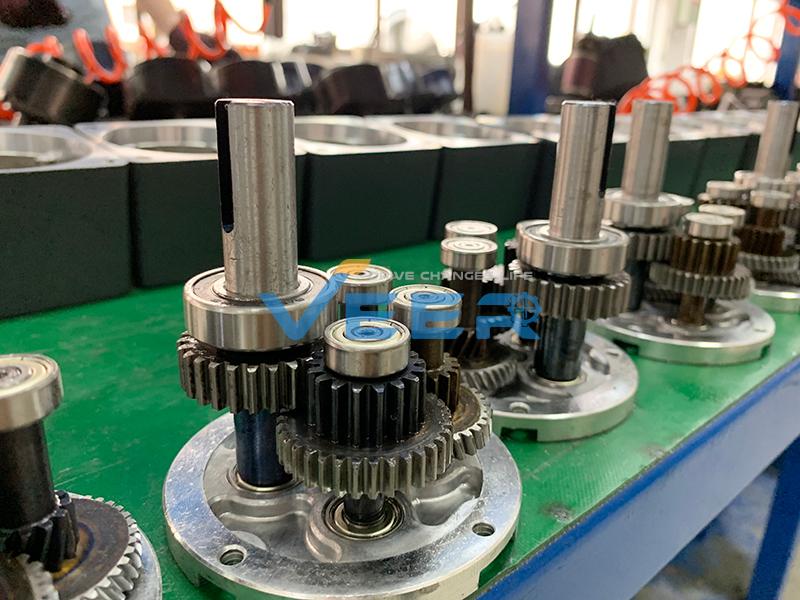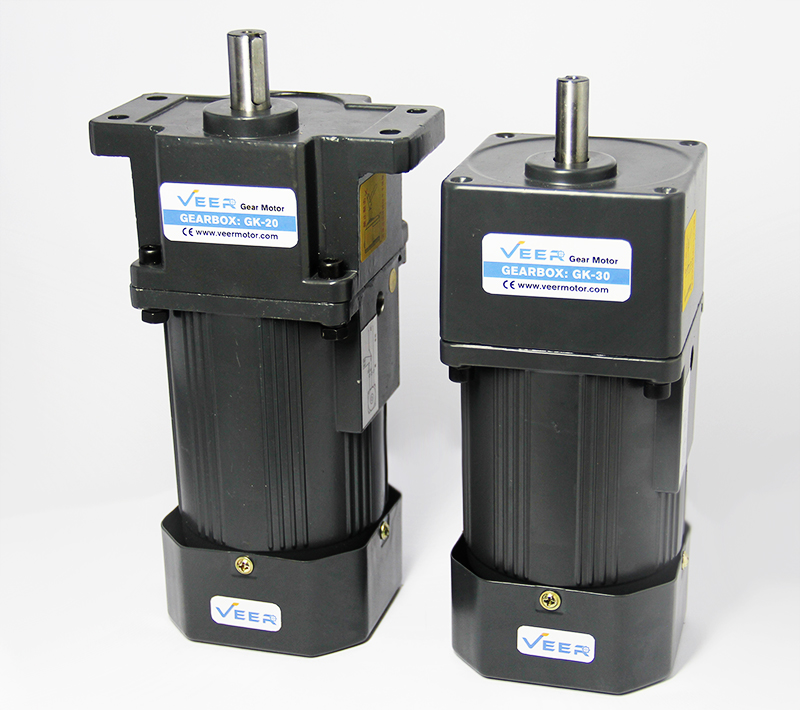# How to detect and prevent vibration caused by gear reduction motor?

If you encounter the vibration from gear reduction motor? Please continue to read as VEER Motor.

•  Whether Speed Reducer (Gearbox) Matches Electric Motor?
The gear reduction motor has both speed index and torque index, the rotate speed and torque are different even the powers of gear reduction motor are same, so as long as the gear motor is adopted by hard connection, there should be considered two indexes (rotate speed and torque). If the torque doesn’t meet the need torque from gear motor that the motor will vibrate or not rotate. Of course, in many times, in cases of rotate speed from high to low, the speed reducer (gearbox) can still running, but there is a defect, if gear motor is running for a long time, which will overload.

• Whether Gear Reduction Motor is overloaded or not?
In general situation, the gear reduction motor is used with other devices during running, the overloads are the needed, and each transmission equipment has their limit load index. So, the limit load index of gear motor should consider all members in whole, and environmental factor, etc. So, load determination is also an important factor to analyze vibration faults.

# How to calculate gear ratio of gear reduction motor?

The “reduction” or gear ratio is calculated by dividing the number of teeth on the large gear by the number of teeth on the small gear. For example, if an electric motor drives a 13-tooth pinion gear that meshes with a 65-tooth gear, a reduction of 5:1 is achieved (65 / 13 = 5). If the electric motor speed is 1,500 rpm, the gearbox reduces this speed by five times to 300 rpm. If the motor torque is 10 lb-in, the gearbox increases this torque by a factor of five to 50 lb-in (before subtracting out gearbox efficiency losses).How to adjust gear motor rotate speed? We are gear motor supplier.